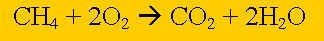Mass-mass stoichiometryMethane burns in oxygen to produce carbon dioxide and water according to the equation above. If 32 grams of methane completely burns in excess oxygen calculate the mass of water formed. Click on the grey boxes for more information on each step. Calculate the amount, in mol, of methane that reacts completely. Step 2 Calculate the ratio from the prefixes in the balanced equation above Step 3 Calculate the number of mole of water produced. Step 4 Calculate the mass of water produced.

Home